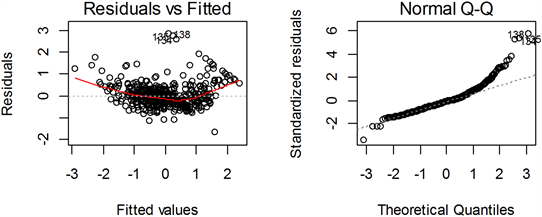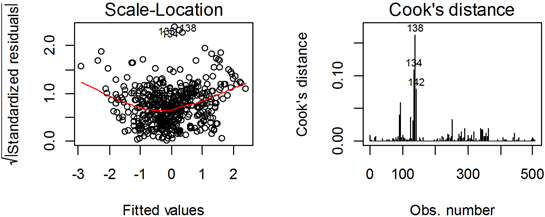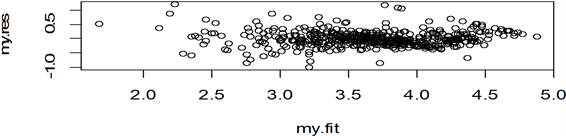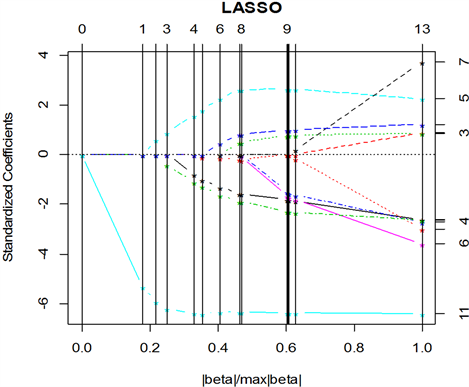# 基于回归方法分析波士顿房价数据间的相关关系Analysis of the Correlation between Housing Price Data in Boston Based on the Regression Method

DOI: 10.12677/SA.2020.93036, PDF, HTML, XML, 下载: 74  浏览: 308

Abstract: According to the variables in the Boston housing price data set, a linear regression model was es-tablished for the Boston housing price by using R software. The significance test of the regression equation and regression coefficient was carried out. The model was established after the Box-Cox transformation was used for the case that the basic assumptions were violated. Lasso regression was used to simplify the equation appropriately, but the regression coefficient of the model estab-lished by lasso regression was small, because the variables in this data were not multicollinearity, which was consistent with the judgment results of R software. Finally, the response variable in the data and the independent variable whose absolute value of its correlation coefficient is greater than 0.5 establish a linear regression equation and predict the housing price. Because the distribution range of housing price in Boston will change with the change of influencing factors, and the median has certain robustness, we establish a regression model for the median of housing price, namely quantile regression model.

1. 引言

2. 材料与方法

2.1. 变量名称与建模目的

2.1.1. 变量名称简介Table 1. Introduction of related variables

2.1.2. 多元线性回归模型的一般形式

$y={\beta }_{0}+{\beta }_{1}{x}_{1}+{\beta }_{2}{x}_{2}+\cdots +{\beta }_{p}{x}_{p}+\epsilon$

$\epsilon$ 是随机误差，并且假定

$\left\{\begin{array}{l}E\left(\epsilon \right)=0\hfill \\ \mathrm{var}\left(\epsilon \right)={\sigma }^{2}\hfill \end{array}$

2.2. 问题解决方法与知识依托

2.2.1. 预处理

${x}_{ij}^{*}=\frac{{x}_{ij}-{\stackrel{¯}{x}}_{j}}{\sqrt{{L}_{jj}/n}},\text{}i=1,2,\cdots ,n;j=1,2,\cdots ,p$

${y}_{i}^{*}=\frac{{y}_{i}-\stackrel{¯}{y}}{\sqrt{{L}_{yy}/n}},\text{}i=1,2,\cdots ,n$

${L}_{jj}=\underset{i=1}{\overset{n}{\sum }}{\left({x}_{ij}-{\stackrel{¯}{x}}_{j}\right)}^{2}$

${\stackrel{^}{y}}^{*}={\stackrel{^}{\beta }}_{1}^{*}{x}_{1}^{*}+{\stackrel{^}{\beta }}_{2}^{*}{x}_{2}^{*}+\cdots +{\stackrel{^}{\beta }}_{p}^{*}{x}_{p}^{*}$

2.2.2. 回归参数的普通最小二乘估计

${\left({X}^{\prime }X\right)}^{-1}$ 存在时，即得回归参数的最小二乘估计为：

$\stackrel{^}{\beta }={\left({X}^{\prime }X\right)}^{-1}{X}^{\prime }y$

2.2.3. 回归方程、回归系数的检验

1) F检验

$F=\frac{SSR/p}{SSE/\left(n-p-1\right)}$

$F>{F}_{\alpha }\left(p,n-p-1\right)$ 时，拒绝原假设 ${H}_{0}$，否则认为在显著性水平 $\alpha$ 下，y与 ${x}_{1},{x}_{2},\cdots ,{x}_{p}$ 有显著的线性关系，即回归方程是显著的。

2) t检验

${H}_{0j}:{\beta }_{j}=0,\text{}j=1,2,\cdots ,p$

${t}_{j}=\frac{\stackrel{^}{\beta }}{\sqrt{{c}_{jj}}\stackrel{^}{\sigma }}$

$\stackrel{^}{\sigma }=\sqrt{\frac{1}{n-p-1}\underset{i=1}{\overset{n}{\sum }}{e}_{i}^{2}}$

2.2.4. 违背基本假设情况的检验

1) 异方差性

$\mathrm{var}\left({\epsilon }_{i}\right)\ne \mathrm{var}\left({\epsilon }_{j}\right)$，当 $i\ne j$

2) 自相关性

$\mathrm{cov}\left({\epsilon }_{i},{\epsilon }_{j}\right)\ne 0$，当 $i\ne j$

2.2.5. 多重共线性

1) 共线性诊断

① 方差扩大因子法

${c}_{jj}=\frac{1}{1-{R}_{j}^{2}}$ 作为方差扩大因子的定义，证明见参考文献 ，当 $VI{F}_{j}\ge 10$ 时，说明自变量 ${x}_{j}$ 与其余自变量之间有严重的多重共线性。(注意：有些教材认为 $vif>4$ 即存在多重共线性。详见参考文献 。)

② 条件数

${X}^{\prime }X$ 的最大特征根为 ${\lambda }_{m}$，称

${k}_{i}=\sqrt{\frac{{\lambda }_{m}}{{\lambda }_{i}}},\text{}i=0,1,\cdots ,p$

2) 解决方法

2.2.6. Lasso回归

Lasso回归又称为套索回归，并提供了从零开始到最小二乘拟合的系数和拟合的整个序列。Lasso是一种收缩估计方法，其基本思想是在回归系数的绝对值之和小于一个常数的约束条件下，使残差平方和最小化，从而能够产生某些严格等于0的回归系数，进一步得到可以解释的模型。R语言中有多个包可以实现Lasso回归，这里使用lars包实现。

3. 结果与分析

3.1. 回归方程的建立

3.1.1. 回归方程的初步建立Table 2. Coefficients of regression equation and their p values

3.1.2. 回归方程的进一步分析

$\begin{array}{c}\stackrel{^}{M}=-0.101C\stackrel{^}{R}+0.116\stackrel{^}{Z}+0.075C\stackrel{^}{H}-0.219\stackrel{^}{N}+0.290RM\\ \text{\hspace{0.17em}}\text{\hspace{0.17em}}-0.342\stackrel{^}{D}+0.284RA-0.216\stackrel{^}{T}-0.223\stackrel{^}{P}+0.092\stackrel{^}{B}-0.406\stackrel{^}{L}\end{array}$

3.2. 违背基本假设情况的检验与解决

$\stackrel{^}{\epsilon }=Y-\stackrel{^}{Y}=\left(I-H\right)Y$

$H=X{\left({X}^{\prime }X\right)}^{-1}{X}^{\prime }$

$\begin{array}{c}y=5.0748-0.01354\text{CRIM}+1.7157\text{e}\text{ }-\text{ }03\text{ZN}+0.1516\text{CHAS}-1.0424\text{NOX}\\ \text{\hspace{0.17em}}\text{\hspace{0.17em}}+0.1422\text{RM}-0.0761\text{DIS}+0.0191\text{RAD}-7.896\text{e}\text{ }-\text{ }04\text{TAX}\\ \text{\hspace{0.17em}}\text{\hspace{0.17em}}-0.0542\text{PTRATIO}+5.917\text{e}\text{ }-\text{ }04\text{B}-0.0397\text{LSTAT}\end{array}$Figure 1. Residual and fitting diagram, QQ diagram, position scale diagram, residual and lever diagram are drawnFigure 2. Comparison of two residuals before and after transformation

Table 3. Regression coefficient after Box-Cox transformation

3.3. 多重共线性的诊断

3.4. 降维

Lasso回归后不为零的回归系数见表4Table 4. Non-zero Lasso regression coefficientFigure 3. Shows the order in which the independent variables are selected

4. 讨论

4.1. 响应变量与部分自变量的回归模型

1) 通过计算自变量与响应变量的相关系数，可以发现与响应变量有较大相关关系的有RM、PTRATIO、LSTAT三个变量，因此对其建立线性回归模型。

$yy=4.889430+0.228333\text{RM}-0.072931\text{PTRATIO}-0.061199\text{LSTAT}$

2) 回归系数的解释

RM增加，MEDV也会增加。因为随着房屋数量的增加，相对房屋价格应该会减小。

LSTAT增加，MEDV会减小。因为低收入者多的地方，他们居住的地区房屋价格会低一些。

PTRATIO增加，MEDV会减小。因为师生数量比表明了一个地方教育发展状况，比值越大，说明该地区缺老师，教育状况较差，因此该地区房价也会低。

4.2. 利用回归模型对自有住房的中位数MEDV进行预测Table 5. Information collected by three customersTable 6. Suggests that the mean of the median house price is

4.3. 模型分析

4.4. 使用性探讨

1978年采集的数据，在考虑通货膨胀的前提下，由于相关的政策发生了变化，因此在今天不适用；

  薛毅, 陈立萍. 统计建模与R软件[M]. 北京: 清华大学出版社, 2007.  周纪芗. 回归分析[M]. 上海: 华东师范大学出版社, 1993.  Kabacoff, R.I. R语言实战[M]. 王小宁, 刘撷芯, 黄俊文, 等, 译. 北京: 人民邮电出版社, 2016: 181.  何晓群, 刘文卿. 应用回归分析[M]. 第5版. 北京: 中国人民大学出版社, 2019.